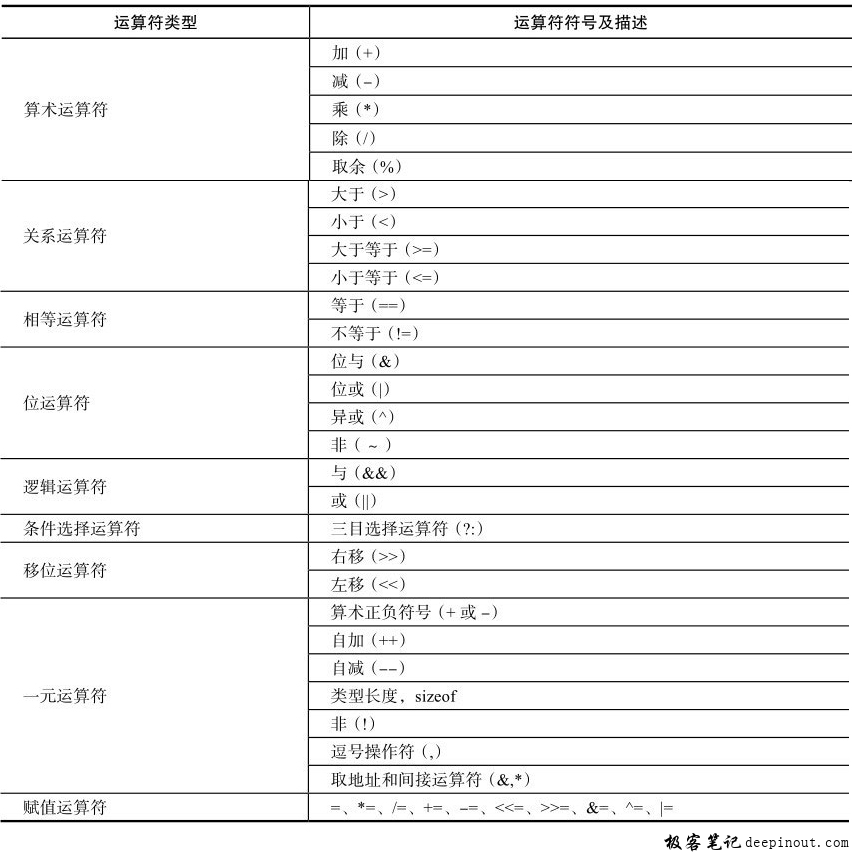# OpenCL 运算符

OpenCL支持的运算符在下表中列出。## 算术运算符

``````int4 vec_a = (int4)(1, 2, 3, 4);
int flag = 3;
int vec_b = vec_a ＊ flag; //vec_b=(1,2,3,4)＊(3,3,3,3)=(3,6,9,12)
``````

## 条件选择运算符

``````int4 vec_a = (int4)(4, 3, 2, 1);
int4 vec_b = (int4)(1, 2, 3, 4);
int4 vec_c = (vec_a > vec_b) ? vec_a : vec_b;
//vec_c.x=(vec_a.x>vec_b.x)?(vec_a.x:vec_b.x)
//vec_c.y=(vec_a.y>vec_b.y)?(vec_a.y:vec_b.y)
//vec_c.z=(vec_a.z>vec_b.z)?(vec_a.z:vec_b.z)
//vec_c.w=(vec_a.w>vec_b.w)?(vec_a.w:vec_b.w)
//vec_c=(4,3,3,4)
vec_a=(in4)(0x8000 0000,100,-1,0);
vec_c=(in4)(-1,-2,-3,-4);
vec_c=vec_a? vec_b:vec_c;
//vec_c=(1,-2,3,-4);
``````

## 一元运算符算术

``````int4 vec_a = (int4)(4, 3, 2, 1);
vec_a++;   //vec_a=(5,4,3,2)
``````

sizeof运算符会得到操作数的字节大小，包含为了对齐而增加的空间。对于标量数据，如果操作数为char或uchar，结果为1；如果操作数为short、ushort或shart，结果为2；如果操作数为int、uint或float，结果为4；如果操作数为long、ulong或double，结果为8。对于n不为3的矢量数据，结果为n*矢量分量大小。而n为3的矢量数据，为了满足对齐要求，结果为4*矢量分量大小。

• 微信订阅
• 回顶